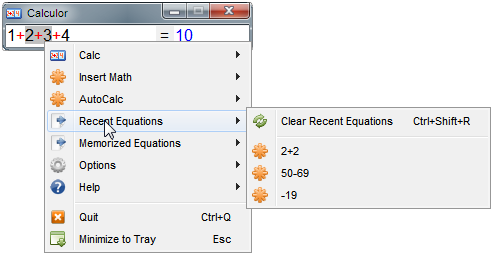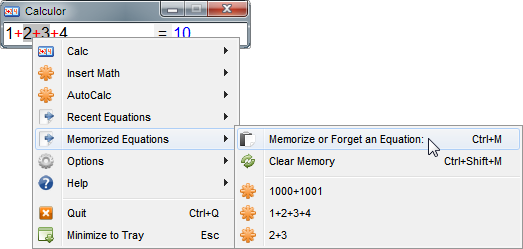﻿ Memory and Recent

# Memory and Recent

Navigation:  »No topics above this level«

# Memory and RecentCalculor has a good memory and can remember lots of things.

There are two ways that Calculor remembers, one is automatically (via Recent Equations) and the other is user-chosen (via Memorized Equations).

He will remember the equation in the equation area every time you Set Answer (usually by pushing the Enter key).

Both the Memory and Recent Lists are preserved when Calculor closes - that means when you run Calculor again, you pick up where you left off.

Recent Equations

You can access this memory in the Recent Equations menu.  You don't need to remember the answers - just the equations - because when you select an entry from the Recent Equations menu, that equation will be put into the equation area and will replace anything you currently have selected.  If nothing is selected it will insert that recent equation at the cursor position.

And, of course, you can use the Clear Recent Equations command to clear the list.

Note: You must use Set Answer (or push the Enter key) in order for an equation to get into the Recent Equations menu.Memorized Equations

But say you have a particular phrase you want to keep ahold of for later.  Select some text in the equation and either push ctrl+M or use the menu and choose Memorize or Forget an Equation.  If the equation isn't already in the Memory list then it will be added.  If it is already there, it will be removed.  And of course, you can remove them all with Clear Memory.